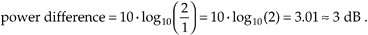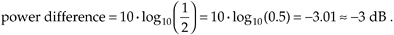# Section E.2. SOME USEFUL DECIBEL NUMBERS

### E 2 SOME USEFUL DECIBEL NUMBERS

If the reader uses dB scales on a regular basis, there are a few constants worth committing to memory. A power difference of 3 dB corresponds to a power factor of 2; that is, if the magnitude-squared ratio of two different frequency components is 2, then from Eq. (E-2),Likewise, if the magnitude-squared ratio of two different frequency components is 1/2, then the relative power difference is –3 dB because

Table E-1. Some seful Logarithmic Relationships

Magnitude ratio

Magnitude-squared power (P1/P2) ratio

Relative dB (approximate)

10–1/2

10–1

–10P1 is one-tenth P2

2–1

2–2 = 1/4

–6P1 is one-fourth P2

2–1/2

2–1 = 1/2

–3P1 is one-half P2

20

20 = 1

0P1 is equal to P2

21/2

21 = 2

3P1 is twice P2

21

22 = 4

6P1 is four times P2

101/2

101 = 10

10P1 is ten times P2

101

102 = 100

20P1 is one hundred times P2

103/2

103 = 1000

30P1 is one thousand times P2

Equation E-9Table E-1 lists several magnitude and power ratios vs. dB values worth remembering. Keep in mind that decibels indicate only relative power relationships. For example, if we're told that signal A is 6 dB above signal B, we know that the power of signal A is four times that of signal B, and that the magnitude of signal A is twice the magnitude of signal B. We may not know the absolute power of signals A and B in watts, but we do know that the power ratio is PA/PB = 4.

URL http://proquest.safaribooksonline.com/0131089897/app05lev1sec2Amazon